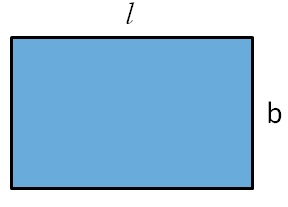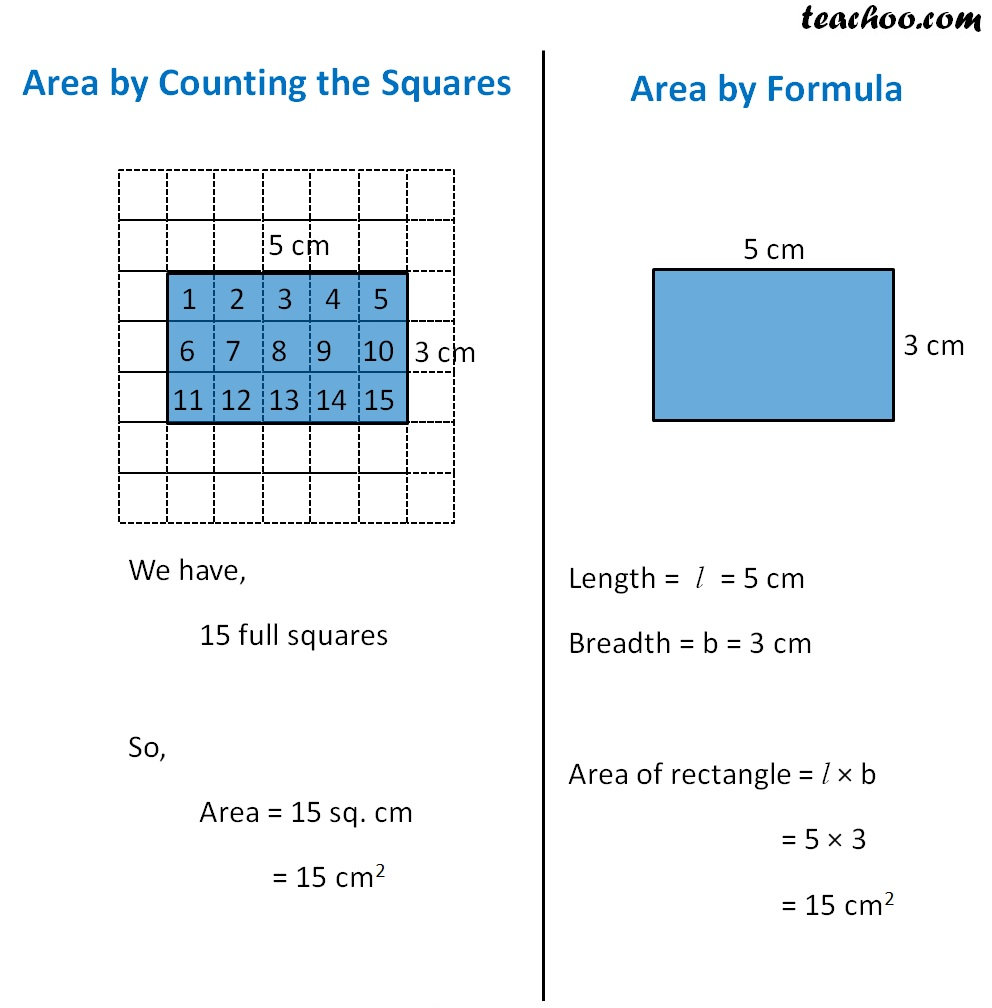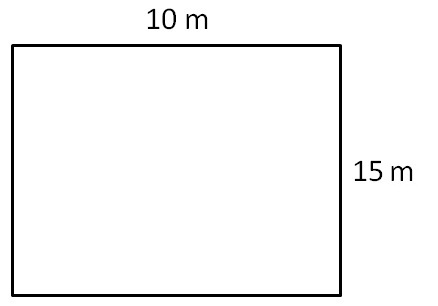Area of Rectangle

Chapter 10 Class 6 Mensuration
Concept wise

We had found area of rectangle by counting the squares

But, that method is too long

To find area of any rectangle,

We use formula

For a rectangle with length l and breadth bArea of rectangle = l × b

## Comparing counting the squares method to formula#### Find area of rectangle with length 10 m and breadth 15 mLength = l = 10 m

Breadth = b = 15 m

Area of rectangle = l × b

= 10 × 15

= 150 sq. m

= 150 m 2

Learn in your speed, with individual attention - Teachoo Maths 1-on-1 Class

### Transcript

We have, 15 full squares So, Area = 15 sq. cm = 15 cm2 Length = l = 5 cm Breadth = b = 3 cm Area of rectangle = l × b = 5 × 3 = 15 cm2